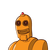# The co-ordinates of the point ofintersection of lines x+2y=3 and 2x-y=1 is *​

The co-ordinates of the point of
intersection of lines x+2y=3 and 2x-
y=1 is *​

### 1 thought on “The co-ordinates of the point of<br />intersection of lines x+2y=3 and 2x-<br />y=1 is *​”

1.(1,1) is the point of intersection

Step-by-step explanation:

x+2y=3 ___________(1)

2x-y=1 _________(2)

Solving (1) and (2) we get the point of intersection

Hope it helps